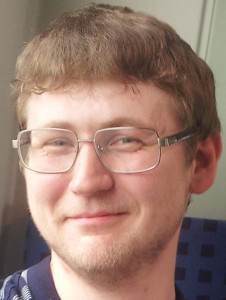# Ivan Novikov, postdocIvan has expertise in mathematical physics, numerical mathematics, optimization methods and mathematical modeling. He graduated from the Department of Problems in Physics and Energetics at the Moscow Institute of Physics and Technology and entered the graduate school of the Institute of Numerical Mathematics of the Russian Academy of Sciences (INM RAS) in 2013. During his graduate studies he was working on the problem of the mean pollution concentration (or economic damage) optimization in Moscow region. Three-dimensional nonstationary convection-diffusion equation, ”standard” initial condition and third type boundary conditions were used as a model of pollution concentration propagation. The problem was formulated in ”the least square sense”, i.e. special quadratic functional including ”controls” (laws utilizing which emission intensities are reduced) was minimized. Ivan developed numerical algorithms for solving the optimization problem mentioned above. Each algorithm guarantees that the mean pollution concentration (or economic damage) defined by sanitary standards is always reached. Moreover, the algorithms proposed allow to calculate ”controls” by using an analytical formula (without an iterative process). Ivan successfully defended his PhD thesis in Mathematical Modelling, Numerical Methods and Complexes of Programs in March 2016.

At Skoltech, Ivan Novikov develops iteratomic interaction models. Using his expertise in numerical mathematics, optimization methods and applying machine learning he constructs models of interatomic interactions with improved efficiency and accuracy. In particular, Ivan’s efforts are focused in the construction of interatomic potentials that predict particle charges in multicomponent structures. The other project Ivan participates in is aimed to prediction of chemical reactions rate coefficients using machine learning (see the details here).

Major publications:

1. Automated Calculation of Thermal Rate Coefficients using Ring Polymer Molecular Dynamics and Machine-Learning Interatomic Potentials with Active Learning (I. Novikov, Y. Suleimanov, A. Shapeev), Physical Chemistry Chemical Physics, 2018, [doi] [arXiv]

2. Improving accuracy of interatomic potentials: more physics or more data? A case study of silica (I.Novikov, A.Shapeev), Materials Today Communications, 2018, [doi] [arXiv]

3. Agoshkov V.I., Novikov I.S. Solution of the pollutants concentration optimization problem with restrictions on intensity of sources // Computational Mathematics and Mathematical Physics, 2016. V. 56, No. 1. P. 26-42.

4. Novikov I.S. Algorithm for solving the problem on pollution risk control related to local sources in region // Russ. J. Numer. Anal. Math. Modelling. 2016. Vol. 31, No. 2. P. 93-103.

5. Novikov I.S. Solving an optimization problem of economic damage from environmental pollution caused by local sources // Numerical Analysis and Applications, 2015. Vol. 8, Iss. 4. P. 329-343.

6. Novikov I.S. Problem of minimization of pollution concentration related to fires in Moscow region // Russ. J. Numer. Anal. Math. Modelling. 2013. Vol. 28, No. 1. P. 13-35.

7. Agoshkov V.I., Aseev N.A., Novikov I.S. The methods of investigation and solution of the problems of local sources and local or integral observations. Moscow: INM RAS. 1st edition, 2012, 151 p. 2nd edition, 2015, 174 p. (in Russian)

8. Agoshkov V.I., Novikov I.S. A problem of minimization pollutants concentration from fires into a region // Ecological safety of coastal and shelf zones and comprehensive use of shelf resources. Collected scientific papers. Iss. 26, vol. 2, Sevastopol, 2012. P. 321-338. (in Russian)

9. Novikov I.S. Algorithms for solving the problem of optimization of the economic impact from environmental pollution that account the resources to eliminate local sources // Computational technologies. 2015. V. 20. No. 4. P. 56-82. (in Russian)